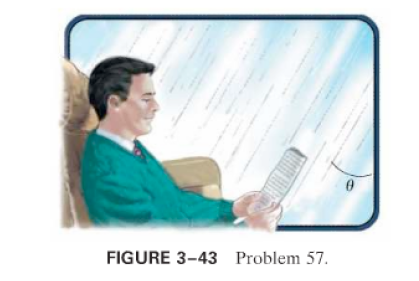×
Get Full Access to Physics: Principles With Applications - 6 Edition - Chapter 3 - Problem 57gp
Get Full Access to Physics: Principles With Applications - 6 Edition - Chapter 3 - Problem 57gp

×

# Raindrops make an angle 8 with the vertical when viewedISBN: 9780130606204 3

## Solution for problem 57GP Chapter 3

Physics: Principles with Applications | 6th Edition

• Textbook Solutions
• 2901 Step-by-step solutions solved by professors and subject experts
• Get 24/7 help from StudySoup virtual teaching assistantsPhysics: Principles with Applications | 6th Edition

4 5 1 384 Reviews
14
0
Problem 57GP

Raindrops make an anglewith the vertical when viewed through a moving train window (Fig.). If the speed of the train is, what is the speed of the raindrops in the reference frame of the Earth in which they are assumed to fall vertically?Step-by-Step Solution:

Problem 57GP

Step-by-step solution

Step 1 of 2

The vector diagram involving the rain and the train are shown below.Step 2 of 2

##### ISBN: 9780130606204

This full solution covers the following key subjects: raindrops, train, speed, angle, fig. This expansive textbook survival guide covers 35 chapters, and 3914 solutions. The answer to “?Raindrops make an angle with the vertical when viewed through a moving train window (Fig. ). If the speed of the train is , what is the speed of the raindrops in the reference frame of the Earth in which they are assumed to fall vertically?” is broken down into a number of easy to follow steps, and 46 words. Physics: Principles with Applications was written by and is associated to the ISBN: 9780130606204. The full step-by-step solution to problem: 57GP from chapter: 3 was answered by , our top Physics solution expert on 03/03/17, 03:53PM. Since the solution to 57GP from 3 chapter was answered, more than 413 students have viewed the full step-by-step answer. This textbook survival guide was created for the textbook: Physics: Principles with Applications, edition: 6.

Unlock Textbook Solution